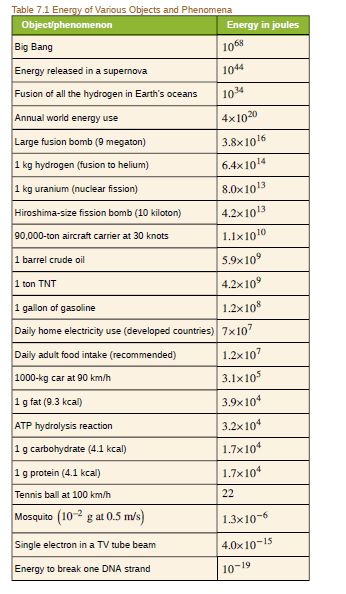Chapter 28, Problem 49PE

Chapter
Section
Textbook Problem

(a) Using data from Table 7.1, calculate the amount of mass converted to energy by the fusion of 1.00 kg of hydrogen. (b) What is the ratio of mass destroyed to the original mass, Δ m / m ? (c) How does this compare with Δ m / m for the fission of 1.00 kg of uranium?(a)

To determine

Kilograms of mass are converted in to energy with the fusion of 1kg hydrogen.

Explanation

Given info:

• The mass of hydrogen is 1kg .
• fusion of 1kg hydrogen produces 6.4×1014Jof energy.

Formula used:

Formula to find mass using Einstein’s mass energy equation,

Δm=E2c

Here, Δm is the converted mass of hydrogen, E is the energy produced and c is the speed of light.

Calculation:

Substituting the given values, we get,

Δm=( 6.4× 10 14 J) (3× 10 8

(b)

To determine

The ratio of the mass destroyed to the original mass.

(c)

To determine

To compare the value of Δmm during fusion of 1kg hydrogen and fission of 1kg uranium.

Still sussing out bartleby?

Check out a sample textbook solution.

See a sample solution

The Solution to Your Study Problems

Bartleby provides explanations to thousands of textbook problems written by our experts, many with advanced degrees!

Get Started